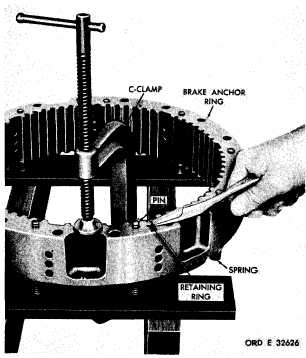Custom SearchCHAP   5,   SEC   X X B R A K E      A N C H O R      R I N G ,      C L U T C H      R E A C T I O N      P L A T E      R E B U I L D PAR  2 - 1 65 Section   XX.   LEFT-   AND   RIGHT-BRAKE   ANCHOR   RING   AND   CLUTCH R E A C T I O N      P L A T E      A S S E M B L I E S — R E B U I LD 1 6 2 .    D E S C R I P T I O N Refer   to   pars.   16   and   17   for   the   descrip- tion   of   the   left-   and   right-brake   anchor   ring and   clutch   reaction   plate   assemblies. 163.   DISASSEMBLY   (figs.   377   and   381, fold-outs   6   and   10) .   L e f t -    a n d    R i g h t - b r a k e    A n c h o r    R i ng A  s  s  e  m  b  l  i  e s .   T h e    l e f t -    a n d    r i g h t - b r a k e anchor   ring   assemblies   are   identical, therefore,    the    following    disassembly procedures   apply   to   either   assembly. (1)   With   the   aid   of   a   C-clamp,   compress the   brake   release   springs,   and   remove   two   re- taining   rings   from   the   brake   return   guide   pins (fig.    207). Figure   207.   Removing   (or   installing)   brake   return guide pin retaining ring ( 2 )    R e m o v e    t h e    g u i d e    p i n s    a n d    r e t u rn springs   (fig.   207). (3)   Remove   the   remaining   seven   retain- ing   rings,   springs   and   guide   pin,   in   the   same manner   as   described   in   (1)   and   (2),   above. .   L e f t -    a n d    R i g h t - c l u t c h    R e a c t i on P l a t e     A s s e m b l i es .   The   left-   and   right-clutch   re- action    plate    assemblies    are    identical, therefore,    the    following    disassembly procedures   apply   to   either   assembly. T h e    f o l l o w i n g    d e s c r i b e s    t h e    d i s a s - sembly of the left-clutch reaction p l a t e    a s s e m b l y . (1)   Do   not   disassemble   clutch   reaction plate   assembly   unless   replacement   of   parts   is n e c e s s a r y .    I f    n e c e s s a r y ,    r e m o v e    t h r e e    s e l f- locking   bolts   72   (fig.   377,   fold-out   6),   using a    l / 2 - i n c h    w r e n c h. ( 2 )    R e m o v e    s l e e v e    7 1. (3)   Remove   two   preformed   packings   70 from   sleeve   71. 1 6 4 .     C L E A N I NG Refer   to   par.   71   for   cleaning   recommen- d a t i o n s. 1 6 5 .    I N S P E C T I O N    A N D    R E P A IR R e f e r    t o    p a r .    7 2    f o r    g e n e r a l    i n s p e c t i on and    repair    recommendations.    Repair    and    re- b u i l d    p o i n t s    o f    m e a s u r e m e n t    f o r    f i t s ,    c l e a r- ances   and   wear   limits   are   indicated   by   small, lower   case   letters   in   figs.   377   and   381,   fold- outs   6   and   10.   Refer   to   pars.   240   and   244   for wear    limits    information. 1 4 2